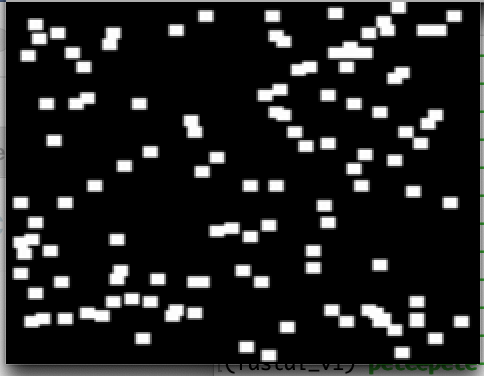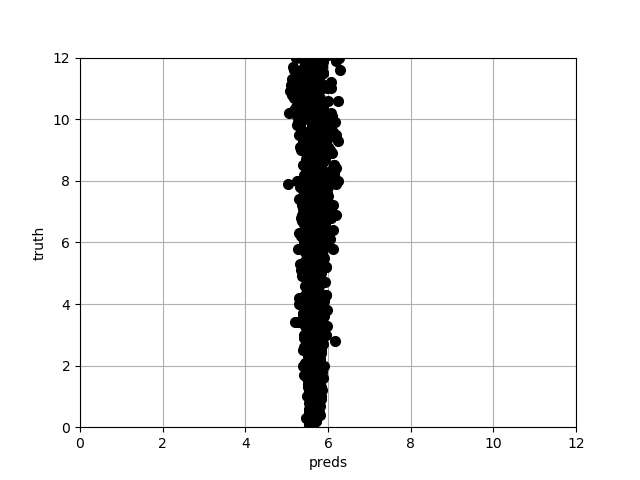# CNN for regression

I’m trying to build a CNN model for regression, with inputs being 1D NumPy arrays.
The problem is that on the test set it just predicts the mean of the set.
In order to try and debug it, I’d like to test the model on a standard dataset. Are you aware of any dataset where inputs are images and the output is a real number? Something that I can load, for instance, from Torchvision.

Hi Lorenzo,

It turns out that I have exactly the same question. I, too, built a net where the last layer outputs a float number, but where the earlier layers contain non-linear elements and convolutions. Puzzled by this, I decided to make a “synthetic” dataset where I generate a number of small rectangles in an image and the “label” is the number of rectangles divided by 10, a float. Below is one of the images.Using my network to process this, I, too, got it converging to the mean. Below is a cross plot of press and truth and the press are gathered about the mean. The cross plot should be an identity mapping = 45 degree line.The head of the model is very similar to what has been discussed in the lesson on bounding boxes, but only one output.

I built my model entirely in raw pytorch in order to make it simple.

I am running out of ideas to fix it. Could it be a Pytorch bug of some sort? Is there some other diagnostic I should do?

If you want, I can give you the simple code that generates the images - but might be simpler just to do it yourself.

BTW one other thing I tried was to make it into a 12 class problem, sacrificing the precision of the floats. This was a little better behaved but, in initial iterations, also got stuck on a single prediction output (not necessarily the mean). I took it to be a local minimum but it could be related to the float problem.

Hi,

I’m using PyTorch too. Actually, I’m trying to reproduce the results of a paper where the authors used Keras. The architecture is not exactly the same but I still believe I’m doing something wrong here. I’ll try to use completely random arrays (both input and output) and check the results.

One other thing about my difficulties: the problem occurs in the training examples as well as the test examples. In fact, it is not possible to overfit (drive the loss to zero) even if I use very few (20) training examples.

I think I have found a work-around. I recalled that in the 2018 course, lesson 9 (I think), Jeremy used a regression on the output head, with 4 floats (not 1 like us). This seemed to work fine in the lesson.

So I made the output 4 floats also, simply by duplicating the label into a vector of length 4. This made my synthetic data converge very well for both train and test.

I then tried it without duplication but simply putting the single float label in a vector of length 1. This also worked! So the problem was that I was generating labels that were not in an array (from the generator - the batches were of course in an array of length batch_size). Apparently, Pytorch cannot handle this properly, but it didn’t complain. I’m not sure if I’d call it a bug but it cost me a bunch of time.

1 Like

Hello All…!! I am relatively new to Machine Learning and I am trying to implement CNN for regression problem. I have an architecture which takes images as input(different scaling factors) and gives a numerical value as an output(determining the scaling factor). The predicted output values do not have enough precision/accuracy compared to the output of the validated data. Why does this happen? How can I improve the performance of my network on the test images? Kindly help me out with this… Cheers.!!